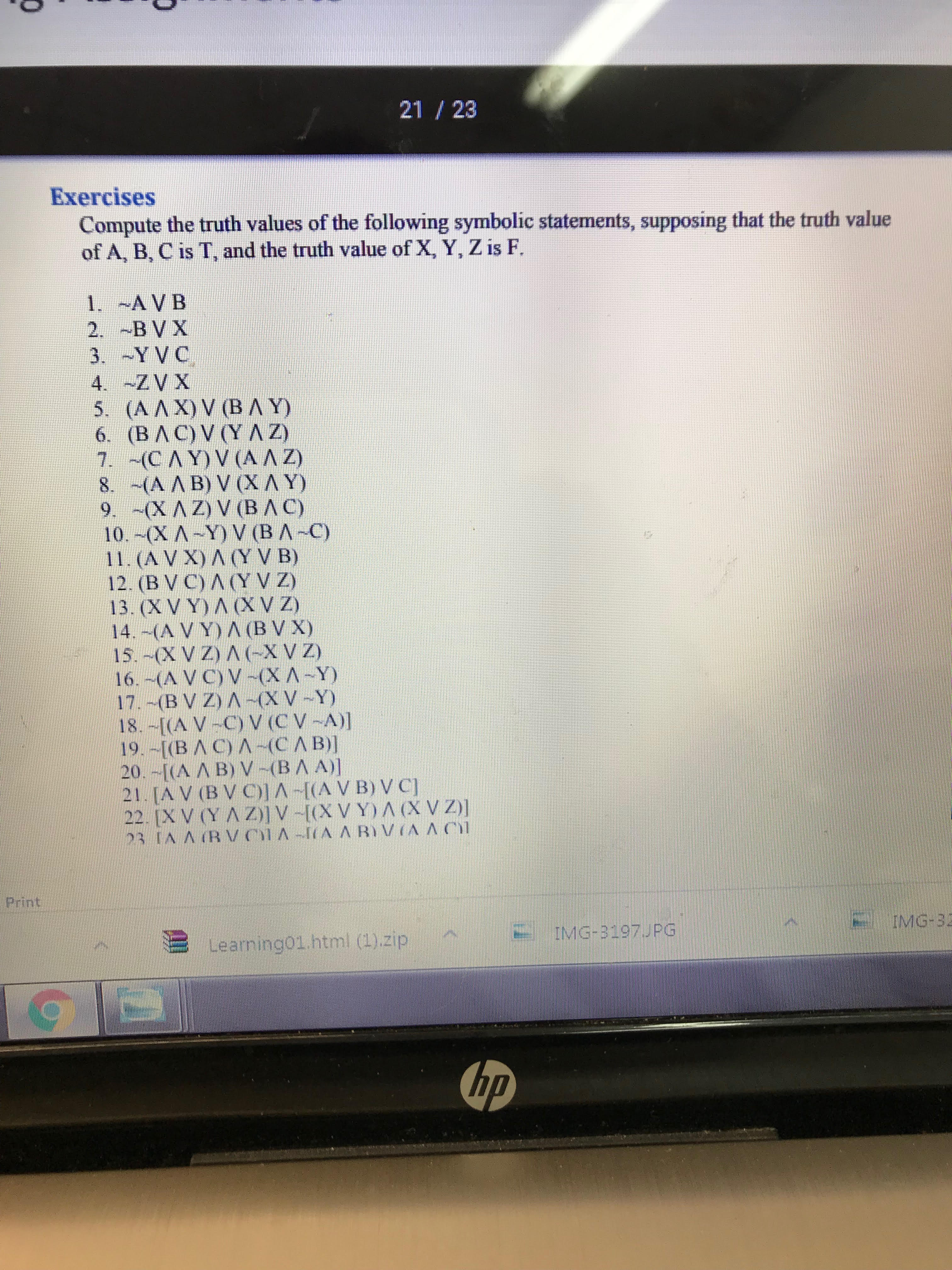# 21 / 23 Exercises Compute the truth values of the following symbolic statements, supposing that the truth value of A, B, C is T, and the truth value of X, Y, Z is F 12.(BVC) Л (YVZ) 13. (X V Y) ACVZ) 16,-(A VC) V-(XA-Y) Print 沺 learning01.html (1).zip.-. 삐 IMG-3197 PG hp

Question

#6help_outlineImage Transcriptionclose21 / 23 Exercises Compute the truth values of the following symbolic statements, supposing that the truth value of A, B, C is T, and the truth value of X, Y, Z is F 12.(BVC) Л (YVZ) 13. (X V Y) ACVZ) 16,-(A VC) V-(XA-Y) Print 沺 learning01.html (1).zip.-. 삐 IMG-3197 PG hp fullscreen

### Want to see the step-by-step answer?